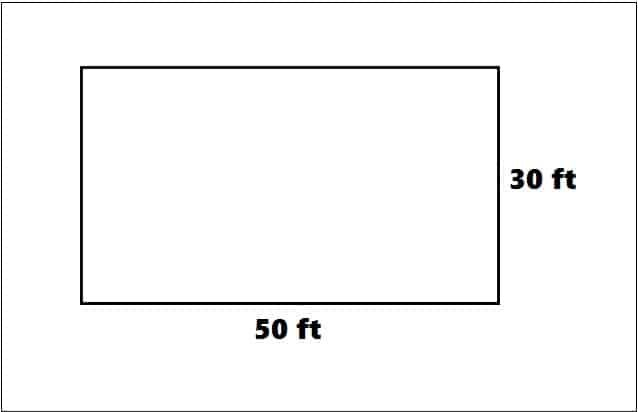# How To Calculate Shuttering Quantity

Contents

## How To Calculate Shuttering Quantity:

Shuttering is an essential temporary structure in which the fresh concrete is poured for casting different members of a structure. The shuttering is removed when concrete achieves its desired strength. There are different materials used in making shuttering such as plywood (as shuttering plate) Nails, wooden battens, beam bottom plates, wooden ballies, shuttering oil etc. In this article, I am going to discuss how to calculate quantity of shuttering by using thumb rule.

### What is Thumb Rule?

Thumb rule is a fixed number determined by our professional experience and daily practice at site. By using thumb rule we get an approximate quantity which is nearly to the exact quantity. Let’s get started.

Calculate shuttering quantity for below given slab:

Type of shuttering – Wooden shutteringThe length of the slab = 50 feet.

The width of the slab = 30 feet.

Total covered area = Length x Width = %0  x 30 = 1500 sft.

### The quantity of Shuttering Required:

#### 1. Plywood:

Generally, the dimension of plywood used in regular construction is 8’x4′ and thickness is 12 mm.

So plywood required = Covered area x Thumb rule = 1500 x 0.02 = 30 no’s

#### 2. Wooden Battens:

Standard dimension of wooden battens is 3″x3″

Wooden battens required = Covered area x Thumb rule = 1500 x 0.04 = 600 rft

rft means running feet, which is the total length of the material. We cut this total length as per our required size.

#### 3. Nails:

Nails required = Covered area x Thumb rule = 1500 x 0.02 = 30 kg.

#### 4. Beam Bottom Plates:

The thickness of beam bottom plate is 1.5″

Beam bottom plates required =  Covered area x Thumb rule = 1500 x 0.24 = 360 rft.

#### 5. Wooden Ballies:

Wooden ballies required for beam = Beam bottom plate running feet x Thumb rule

= 360 x 0.5 = 180 no’s

Wooden ballies required for slab = Covered area x Thumb rule = 1500 x 0.16

= 1500 x 0.16 =240 no’s

#### 6. MS Clamps:

MS Clamps required = Covered area x Thumb rule 1500 x 0.08 = 120 no’s

#### 7. Shuttering Oil:

Shuttering oil required = Covered area x Thumb rule = 1500 x 0.006 = 9 liters

Note:

1. These are just approx quantity. To get exact quantity you need to get the exact dimension of members where the shuttering will be used.

2. The above thumb rules may vary with changing the dimension of shuttering plate etc.

### 5 thoughts on “How To Calculate Shuttering Quantity”

1. Nice information, Good